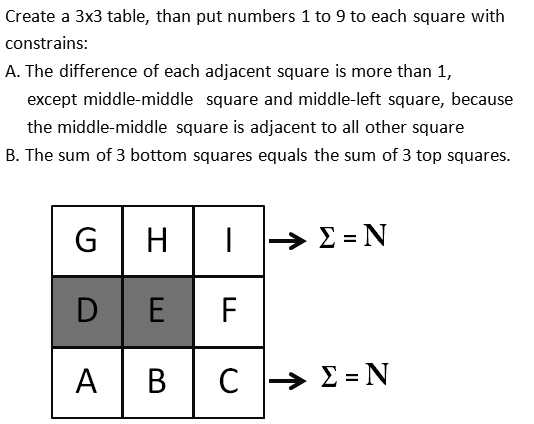BrainDen.com - Brain Teasers
• 0

# 3 x 3 table with constrains

## QuestionThere are 2 solutions, if we rule out Reflection

Edited by jasen

## Recommended Posts

• 1
1 hour ago, jasen said:

Reflection works vertically

Ah, I missed a few of the diagonals (I accounted for the center square but not all):

Spoiler

2 unique solutions, 1 reflection per solution (across the X-Axis)

A=1, B=5, C=7, D=8, E=9, F=2, G=3, H=6, I=4       (G+H+I) = (A+B+C) =13
A=3, B=6, C=4, D=8, E=9, F=2, G=1, H=5, I=7       (G+H+I) = (A+B+C) =13

A=7, B=4, C=6, D=2, E=1, F=8, G=9, H=5, I=3       (G+H+I) = (A+B+C) =17
A=9, B=5, C=3, D=2, E=1, F=8, G=7, H=4, I=6       (G+H+I) = (A+B+C) =17

##### Share on other sites

• 0
Spoiler

So reflections/rotations get a little strange with this because of the fact that you have stated that the middle-left and middle-middle squares can be a difference of 1. This means if you have a solution that works...and then reflect it across the Y-axis, there's a possibility that that reflected solution is not a valid solution anymore. Here's an example of this:

This solution works with the original configuration:

A=1, B=5, C=7, D=8, E=9, F=2, G=3, H=6, I=4       (G+H+I) = (A+B+C) =13

If I reflect that across the Y-axis...you get this (which is no longer valid because E/F have a difference one 1):

A=7, B=5, C=1, D=2, E=9, F=8, G=4, H=6, I=3       (G+H+I) = (A+B+C) =13

With that being said, I could spend the time figuring out which ones are the true reflections/rotations/duplicates (some are easy to figure out) and which ones aren't...but here's all 24 of the possible solutions to the above:

A=1, B=5, C=7, D=8, E=9, F=2, G=3, H=6, I=4       (G+H+I) = (A+B+C) =13
A=1, B=7, C=3, D=8, E=9, F=6, G=5, H=2, I=4       (G+H+I) = (A+B+C) =11
A=1, B=7, C=5, D=8, E=9, F=2, G=3, H=6, I=4       (G+H+I) = (A+B+C) =13
A=3, B=6, C=4, D=8, E=9, F=2, G=1, H=5, I=7       (G+H+I) = (A+B+C) =13
A=3, B=6, C=4, D=8, E=9, F=2, G=1, H=7, I=5       (G+H+I) = (A+B+C) =13
A=3, B=6, C=4, D=8, E=9, F=2, G=5, H=1, I=7       (G+H+I) = (A+B+C) =13
A=3, B=7, C=1, D=8, E=9, F=6, G=5, H=2, I=4       (G+H+I) = (A+B+C) =11
A=3, B=7, C=2, D=8, E=9, F=4, G=5, H=1, I=6       (G+H+I) = (A+B+C) =12
A=5, B=1, C=6, D=8, E=9, F=4, G=3, H=7, I=2       (G+H+I) = (A+B+C) =12
A=5, B=1, C=7, D=8, E=9, F=2, G=3, H=6, I=4       (G+H+I) = (A+B+C) =13
A=5, B=2, C=4, D=8, E=9, F=6, G=1, H=7, I=3       (G+H+I) = (A+B+C) =11
A=5, B=2, C=4, D=8, E=9, F=6, G=3, H=7, I=1       (G+H+I) = (A+B+C) =11
A=5, B=8, C=6, D=2, E=1, F=4, G=7, H=3, I=9       (G+H+I) = (A+B+C) =19
A=5, B=8, C=6, D=2, E=1, F=4, G=9, H=3, I=7       (G+H+I) = (A+B+C) =19
A=5, B=9, C=3, D=2, E=1, F=8, G=7, H=4, I=6       (G+H+I) = (A+B+C) =17
A=5, B=9, C=4, D=2, E=1, F=6, G=7, H=3, I=8       (G+H+I) = (A+B+C) =18
A=7, B=3, C=8, D=2, E=1, F=6, G=5, H=9, I=4       (G+H+I) = (A+B+C) =18
A=7, B=3, C=9, D=2, E=1, F=4, G=5, H=8, I=6       (G+H+I) = (A+B+C) =19
A=7, B=4, C=6, D=2, E=1, F=8, G=5, H=9, I=3       (G+H+I) = (A+B+C) =17
A=7, B=4, C=6, D=2, E=1, F=8, G=9, H=3, I=5       (G+H+I) = (A+B+C) =17
A=7, B=4, C=6, D=2, E=1, F=8, G=9, H=5, I=3       (G+H+I) = (A+B+C) =17
A=9, B=3, C=5, D=2, E=1, F=8, G=7, H=4, I=6       (G+H+I) = (A+B+C) =17
A=9, B=3, C=7, D=2, E=1, F=4, G=5, H=8, I=6       (G+H+I) = (A+B+C) =19
A=9, B=5, C=3, D=2, E=1, F=8, G=7, H=4, I=6       (G+H+I) = (A+B+C) =17

##### Share on other sites

• 0

"the middle-middle square is adjacent to all other square"

This implies that the restriction applies to diagonally adjacent squares. There are only two solutions, along with their vertical reflections, that qualify in this case.

##### Share on other sites

• 0
21 hours ago, Pickett said:
Reveal hidden contents

So reflections/rotations get a little strange with this because of the fact that you have stated that the middle-left and middle-middle squares can be a difference of 1. This means if you have a solution that works...and then reflect it across the Y-axis, there's a possibility that that reflected solution is not a valid solution anymore. Here's an example of this:

This solution works with the original configuration:

------ cut  ----

Reflection works vertically

Edited by jasen

## Join the conversation

You can post now and register later. If you have an account, sign in now to post with your account.×   Pasted as rich text.   Paste as plain text instead

Only 75 emoji are allowed.

×   Your previous content has been restored.   Clear editor

×   You cannot paste images directly. Upload or insert images from URL.